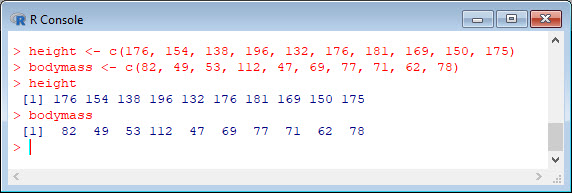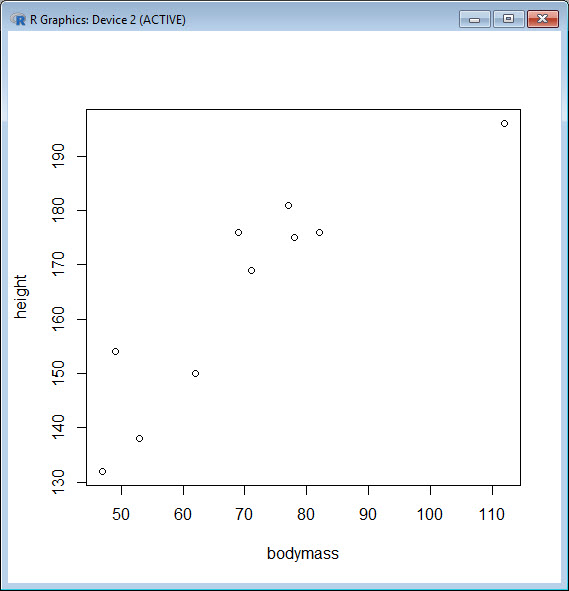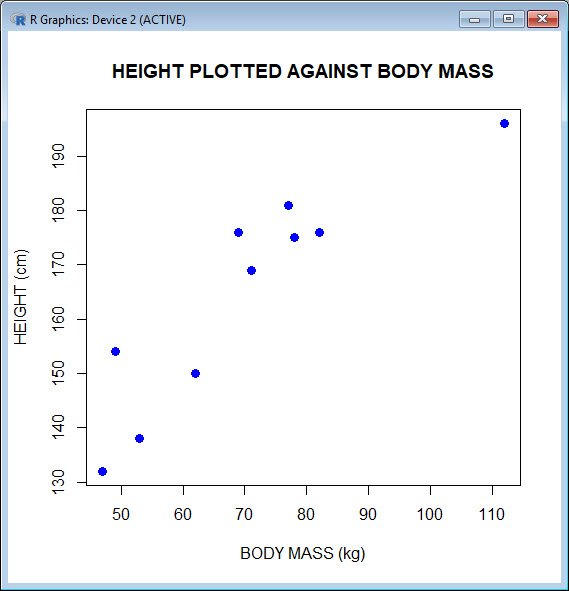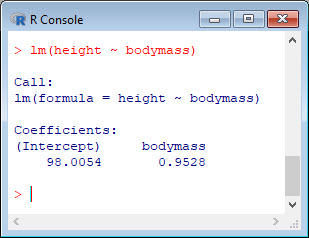Today let’s re-create two variables and see how to plot them and include a regression line. We take height to be a variable that describes the heights (in cm) of ten people. Copy and paste the following code to the R command line to create this variable.
`height <- c(176, 154, 138, 196, 132, 176, 181, 169, 150, 175)`
Now let’s take bodymass to be a variable that describes the masses (in kg) of the same ten people. Copy and paste the following code to the R command line to create the weight variable.
`bodymass <- c(82, 49, 53, 112, 47, 69, 77, 71, 62, 78)`
Both variables are now stored in the R workspace. To view them, enter:
```height bodymass ```We can now create a simple plot of the two variables as follows:
`plot(bodymass, height)`We can enhance this plot using various arguments within the `plot()` command. Copy and paste the following code into the R workspace:
`plot(bodymass, height, pch = 16, cex = 1.3, col = "blue", main = "HEIGHT PLOTTED AGAINST BODY MASS", xlab = "BODY MASS (kg)", ylab = "HEIGHT (cm)")`In the above code, the syntax `pch = 16` creates solid dots, while `cex = 1.3` creates dots that are 1.3 times bigger than the default (where `cex = 1`). More about these commands later.
Now let’s perform a linear regression using `lm()` on the two variables by adding the following text at the command line:
`lm(height ~ bodymass)`We see that the intercept is 98.0054 and the slope is 0.9528. By the way – `lm` stands for “linear model”.
Finally, we can add a best fit line (regression line) to our plot by adding the following text at the command line:
`abline(98.0054, 0.9528)`
Another line of syntax that will plot the regression line is:
`abline(lm(height ~ bodymass))`None of this was so difficult! In our next blog we will look again at regression.
David

#### Annex: R codes used

```# Create the height variable.
height <- c(176, 154, 138, 196, 132, 176, 181, 169, 150, 175)

# Create the weight variable.
bodymass <- c(82, 49, 53, 112, 47, 69, 77, 71, 62, 78)

# View both variables.
height
bodymass

# Create a scatterplot of height against bodymass variable.
plot(bodymass, height)

# Create more complete scatterplot of height against bodymass variable.
plot(bodymass, height, pch = 16, cex = 1.3, col = "blue", main = "HEIGHT PLOTTED AGAINST BODY MASS", xlab = "BODY MASS (kg)", ylab = "HEIGHT (cm)")

# Perform a linear regression on the two variables.
lm(height ~ bodymass)

# Add a best fit line (regression line) to the plot.
abline(98.0054, 0.9528)

# Alternatively we can plot the regression line using the following syntax:
abline(lm(height ~ bodymass))
```